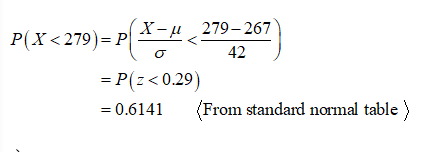# Suppose that the distance of fly balls hit to the outfield (in baseball) is normally distributed with a mean of 267 feet and a standard deviation of 42 feet. Let X be the distance in feet for a fly ball.a. What is the distribution of X? X ~ Nb. Find the probability that a randomly hit fly ball travels less than 279 feet. Round to 4 decimal placesc. Find the 90th percentile for the distribution of distance of fly balls. Round to 2 decimal places.

Question
2 views

Suppose that the distance of fly balls hit to the outfield (in baseball) is normally distributed with a mean of 267 feet and a standard deviation of 42 feet. Let X be the distance in feet for a fly ball.

a. What is the distribution of X? X ~ N

b. Find the probability that a randomly hit fly ball travels less than 279 feet. Round to 4 decimal places

c. Find the 90th percentile for the distribution of distance of fly balls. Round to 2 decimal places.

check_circle

Step 1

a)

From the given information, the distribution of the distance of fly balls hit to the outfield follows normal distribution with mean of 267 and standard deviation of 42.

Step 2

Therefore, the distribution of X follows N (267, 42).

b)

The probability that a randomly hit fly ball travels less than 279 feet is obtained as follows:...

### Want to see the full answer?

See Solution

#### Want to see this answer and more?

Solutions are written by subject experts who are available 24/7. Questions are typically answered within 1 hour.*

See Solution
*Response times may vary by subject and question.
Tagged in

### Statistics